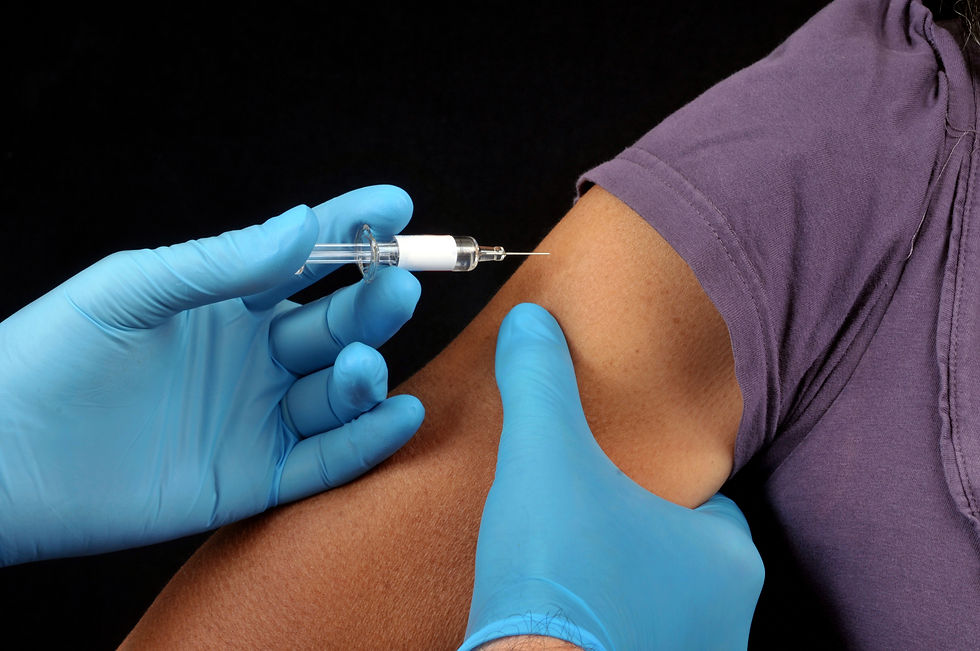# Dr. Muhammad Altaf Khan (City University of Science & Information Technology Peshawar, Pakistan)Dr. Muhammad Altaf Khan (City University of Science & Information Technology Peshawar, Pakistan)​ is co-author of a recent paper which presents a deterministic model for dengue virus transmission and aims to investigate the optimal strategy for curtailing the spread of dengue. The model is parameterized using data from the 2017 dengue outbreak in Pakistan, uses optimal control theory and includes two time-dependent control variables determined from sensitivity analysis, insecticide use and vaccination. The results show that the two controls avert the same number of infections in the district considered regardless of the weights on the costs of insecticide use and vaccination, due to the reciprocal relationship between the cost of insecticide use and vaccination.

How was the optimal control theory applied in your model to investigate the best strategy for curtailing the spread of dengue?

The Optimal Control Theory is a powerful technique and it has uses for control systems not only in mathematical models of infectious diseases but also in engineering problems. In this case, we first formulated the model with vaccination and then without vaccination. Then, we evaluated the most appropriate controls for the model by checking the sensitivity of parameters. The sensitivity analysis of the model parameters and its effect on the basic reproduction number shows the possible strategy for dengue elimination.

The results of the sensitivity analysis of the model suggest that a control strategy willadequately reduce the spread of dengue in the community if it reduces the following components: the transmission probability per contact of infectious mosquitoes with susceptible humans, the mosquito recruitment rate, the mosquito biting rate, the transmission probability per contact of susceptible mosquitoes with infectious humans, disease progression rate in mosquitoes, and vaccine waning rate.

Furthermore, a strategy that increases the human recruitment rate, disease progression rate in humans, human recovery rate mosquito natural death rate, and vaccine efficacy will be effective in curtailing the spread of dengue in the community.

Therefore, we introduced into the transmission model a time-dependent control variable the vaccination rate, which was previously taken as a constant. Although this parameter does not significantly impact the reproduction number, we nevertheless consider this parameter as a time-dependent variable since the vaccine efficacy has a significant impact on the basic reproduction number.We also considered another control variable for the mosquito population since the mosquito death rate showed a strong significant impact on the basic reproduction number. The mosquito biting rate and transmission probabilities such as the transmission probability from exposed mosquitoes to susceptible humans, transmission probability from infected mosquitoes to susceptible humans, transmission probability from exposed humans to susceptible mosquitoes, transmission probability from infected human to susceptible mosquitoes are another set of candidate parameters to use as a time dependent controls. Based on these results we modelled the controls for dengue elimination.

Could you elaborate on the modelling you used?